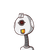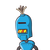# find the area of a rectangle whose length and breadth are 25 cm and 15 cm respectively.​

find the area of a rectangle whose length and breadth are 25 cm and 15 cm respectively.​

### 2 thoughts on “find the area of a rectangle whose length and breadth are 25 cm and 15 cm respectively.​”

1.Step-by-step explanation:

area of rectangle is length * breadth

= 25 * 15

= 375 CM sq

2.Step-by-step Explanation:

To find the area of rectangle we have to put the value in their place and apply the formula..

here the following steps to find the area of rectangle :

Area of Rectangle = (Length × breadth)

Area of Rectangle = (25×15) cm

Area of Rectangle = 375cm²

Hope it will helps you...